# 17 Python数据库编程(Win版)

## 17.1 相关数据库软件安装

### 17.1.1 MySQL服务器的安装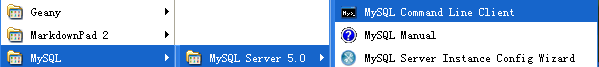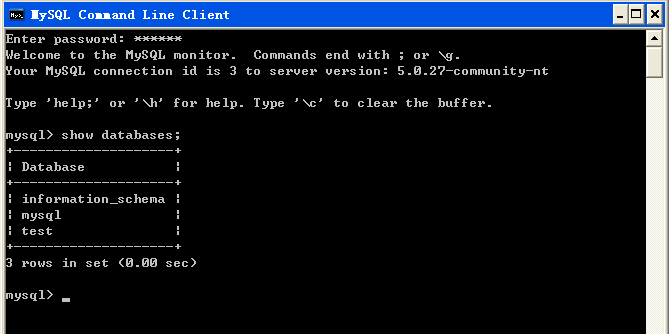## 17.2 MySQL数据库的基本使用

WindowsXP上安装好了MySQL-Server以后，便可通过MySQL的客户端登录MySQL服务器，在服务器上创建数据库、数据表并对表进行插入、查询等操作。

### 操作使用某库

MySQL数据库，修改的是库下的表，为此得首先打开库，使用命令为`use 某数据库名;`，现在的想法是在新建的cpython数据库里建一个表，得首先使用这个cpython库，操作命令为`use cpython;`，如下图所示：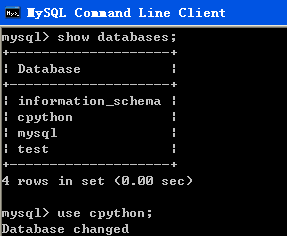### 创建表

cpython库为刚刚创建的新的数据库，其下目前是没有任何表的，可以用一下`show tables;`来看看。那好，那就创建一个表吧名字为user的表，命令如下： `create table user(id int, name varchar(32));`，实际操作截图如下所示：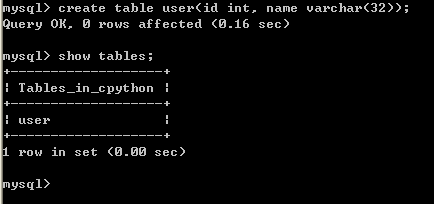### 查看表里的数据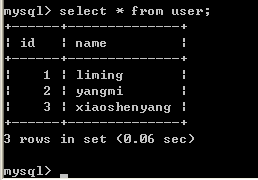## 17.3 Python访问MySQL数据库

Python可以通过MySQLdb模块编程访问MySQL数据库里的某库下的某表，进行对表的增删改查等操作。MySQLdb模块在windows下是通过本章的17.2节安装，其他系统平台例如Linux计算机安装MySQLdb相当简单，一条命令即可完成。

``````\$ sudo pip install mysql-python
``````

### 17.3.1 Python访问MySQL编程步骤

#### 17.3.1.1 建立数据库服务器连接访问连接对象

``````import MySQLdb
conn = MySQLdb.connect(host = "localhost", user = "root", passwd = "123456", db = "cpython")
``````

#### 17.3.1.2 建立 python 和数据库服务器交互的游标对象

``````cur = conn.cursor()
``````

#### 如果是查询，可以通过游标从服务器获得结果集

``````sql = "select * from user;"
cur.execute(sql)
ret = cur.fetchall()
``````

#### 如果是插入或修改，在提交sql后还需通过连接对象修改数据库或表

``````sql = "insert into user values(3, 'reliya');"
cur.execute(sql)
conn.commit()
``````

### 17.3.2 Python访问数据库MySQL完整程序

#### 示例1：读取cpython数据库user表里的数据

``````import MySQLdb
conn = MySQLdb.connect(host = "localhost", user = "root", passwd = "123456", db = "cpython")
cur = conn.cursor()
sql = "select * from user;"
cur.execute(sql)
ret = cur.fetchall()
for x in ret:
print x
cur.close()
conn.close()
``````

``````(1L, 'liming')
(2L, 'yangmi')
(3L, 'xiaoshenyang')
``````

#### 示例2：提交新数据

``````import MySQLdb
import random
conn = MySQLdb.connect(host = "localhost", user = "root", passwd = "123456", db = "cpython")
cur = conn.cursor()
x = random.randint(1, 100)
sql = "insert into user values(%d, '%s');" %(x, "cpython" + str(x))
cur.execute(sql)
conn.commit()
sql = "select * from user;"
cur.execute(sql)
ret = cur.fetchall()
for x in ret:
print x
cur.close()
conn.close()
``````

``````(1L, 'liming')
(2L, 'yangmi')
(3L, 'xiaoshenyang')
(41L, 'cpython41')
(11L, 'cpython11')
(97L, 'cpython97')
(11L, 'cpython11')
``````